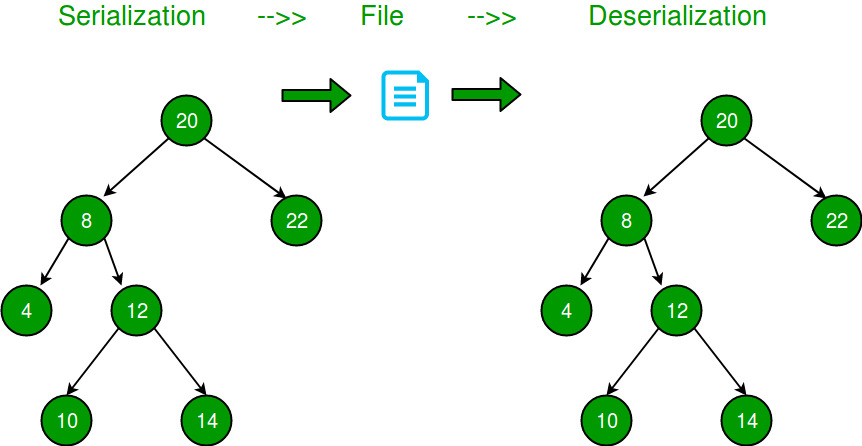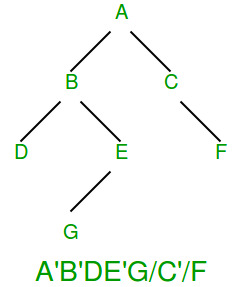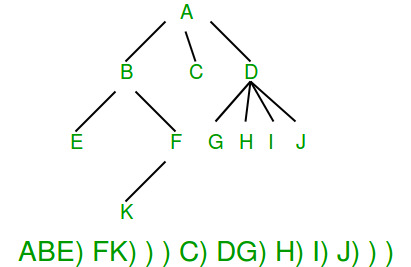# Serialize and Deserialize a Binary Tree

Serialization is to store the tree in a file so that it can be later restored. The structure of the tree must be maintained. Deserialization is reading the tree back from a file.Following are some simpler versions of the problem:

## If given Tree is a Binary Search Tree?

If the given Binary Tree is Binary Search Tree, we can store it by either storing preorder or postorder traversal. In case of Binary Search Trees, only preorder or postorder traversal is sufficient to store structure information

## If given Binary Tree is Complete Tree?

A Binary Tree is complete if all levels are completely filled except possibly the last level and all nodes of last level are as left as possible (Binary Heaps are complete Binary Tree). For a complete Binary Tree, level order traversal is sufficient to store the tree. We know that the first node is root, next two nodes are nodes of next level, next four nodes are nodes of 2nd level and so on.

## If given Binary Tree is Full Tree?

A full Binary is a Binary Tree where every node has either 0 or 2 children. It is easy to serialize such trees as every internal node has 2 children. We can simply store preorder traversal and store a bit with every node to indicate whether the node is an internal node or a leaf node.

## How to store a general Binary Tree?

A simple solution is to store both Inorder and Preorder traversals.

This solution requires space twice the size of the Binary Tree. We can save space by storing Preorder traversal and a marker for NULL pointers.

• Store all possible child nodes for each node.
• If there is no child node then push -1 for that child.
• Put this preorder traversal in the file.

Examples:

Input:
12
/
13
Output: 12 13 -1 -1 -1

Input:
20
/   \
8     22
Output: 20 8 -1 -1 22 -1 -1

Input:
20
/
8
/  \
4   12
/   \
10  14
Output: 20 8 4 -1 -1 12 10 -1 -1 14 -1 -1 -1

Input:
20
/
8
/
10
/
5
Output: 20 8 10 5 -1 -1 -1 -1 -1

Input:
20
\
8
\
10
\
5

Output: 20 -1 8 -1 10 -1 5 -1 -1

## How to deserialize the above serialization?

Deserialization can be done by simply reading data from the file one by one and keep on adding children till a -1 is reached. If both the children are NULL then return back to the parent.

Below is the implementation of the above idea.

## C++

 `// A C++ program to demonstrate serialization and` `// deserialization of Binary Tree`   `#include ` `using` `namespace` `std;`   `#define MARKER -1`   `// A binary tree Node has key, ` `// pointer to left and right children` `struct` `Node {` `    ``int` `key;` `    ``struct` `Node *left, *right;` `};`   `// Helper function that allocates a new Node with the` `// given key and NULL left and right pointers.` `struct` `Node* newNode(``int` `key)` `{` `    ``struct` `Node* temp = ``new` `Node();` `    ``temp->key = key;` `    ``temp->left = temp->right = NULL;` `    ``return` `(temp);` `}`   `// This function stores a tree in a file pointed by fp` `void` `serialize(Node* root, ``FILE``* fp)` `{` `    ``// If current node is NULL, store marker` `    ``if` `(root == NULL) {` `        ``fprintf``(fp, ``"%d "``, MARKER);` `        ``return``;` `    ``}`   `    ``// Else, store current node and ` `    ``// recur for its children` `    ``fprintf``(fp, ``"%d "``, root->key);` `    ``serialize(root->left, fp);` `    ``serialize(root->right, fp);` `}`   `// This function constructs a tree from ` `// a file pointed by 'fp'` `void` `deSerialize(Node*& root, ``FILE``* fp)` `{` `    ``// Read next item from file. ` `    ``// If there are no more items` `    ``// or next item is marker, then return` `    ``int` `val;` `    ``if` `(!``fscanf``(fp, ``"%d "``, &val) || val == MARKER)` `        ``return``;`   `    ``// Else create node with this item ` `    ``// and recur for children` `    ``root = newNode(val);` `    ``deSerialize(root->left, fp);` `    ``deSerialize(root->right, fp);` `}`   `// A simple inorder traversal used for ` `// testing the constructed tree` `void` `inorder(Node* root)` `{` `    ``if` `(root) {` `        ``inorder(root->left);` `        ``printf``(``"%d "``, root->key);` `        ``inorder(root->right);` `    ``}` `}`   `// Driver code` `int` `main()` `{` `    ``// Construct a tree shown in the above figure` `    ``struct` `Node* root = newNode(20);` `    ``root->left = newNode(8);` `    ``root->right = newNode(22);` `    ``root->left->left = newNode(4);` `    ``root->left->right = newNode(12);` `    ``root->left->right->left = newNode(10);` `    ``root->left->right->right = newNode(14);`   `    ``// Open a file and serialize the tree into the file` `    ``FILE``* fp = ``fopen``(``"tree.txt"``, ``"w"``);` `    ``if` `(fp == NULL) {` `        ``puts``(``"Could not open file"``);` `        ``return` `0;` `    ``}` `    ``serialize(root, fp);` `    ``fclose``(fp);`   `    ``// Deserialize the stored tree into root1` `    ``Node* root1 = NULL;` `    ``fp = ``fopen``(``"tree.txt"``, ``"r"``);` `    ``deSerialize(root1, fp);`   `    ``printf``(``"Inorder Traversal of the tree constructed from "` `           ``"file:\n"``);` `    ``inorder(root1);`   `    ``return` `0;` `}`

## Java

 `// A Java program to demonstrate serialization and` `// deserialization of Binary Tree`   `import` `java.util.*;`   `// A binary tree Node has key,` `// pointer to left and right children` `class` `TreeNode {` `    ``int` `val;` `    ``TreeNode left;` `    ``TreeNode right;` `    ``TreeNode(``int` `x) { val = x; }` `}`   `class` `BinaryTree {` `    ``TreeNode root;`   `    ``// Encodes a tree to a single string.` `    ``public` `static` `String serialize(TreeNode root)` `    ``{` `        ``if` `(root == ``null``) {` `            ``return` `null``;` `        ``}` `        ``Stack s = ``new` `Stack<>();` `        ``s.push(root);`   `        ``List l = ``new` `ArrayList<>();` `        ``while` `(!s.isEmpty()) {` `            ``TreeNode t = s.pop();`   `            ``// If current node is NULL, store marker` `            ``if` `(t == ``null``) {` `                ``l.add(``"#"``);` `            ``}` `            ``else` `{`   `                ``// Else, store current node` `                ``// and recur for its children` `                ``l.add(``""` `+ t.val);` `                ``s.push(t.right);` `                ``s.push(t.left);` `            ``}` `        ``}` `        ``return` `String.join(``","``, l);` `    ``}`   `    ``static` `int` `t;`   `    ``// Decodes your encoded data to tree.` `    ``public` `static` `TreeNode deserialize(String data)` `    ``{` `        ``if` `(data == ``null``)` `            ``return` `null``;` `        ``t = ``0``;` `        ``String[] arr = data.split(``","``);` `        ``return` `helper(arr);` `    ``}`   `    ``public` `static` `TreeNode helper(String[] arr)` `    ``{` `        ``if` `(arr[t].equals(``"#"``))` `            ``return` `null``;`   `        ``// Create node with this item` `        ``// and recur for children` `        ``TreeNode root` `            ``= ``new` `TreeNode(Integer.parseInt(arr[t]));` `        ``t++;` `        ``root.left = helper(arr);` `        ``t++;` `        ``root.right = helper(arr);` `        ``return` `root;` `    ``}`   `    ``// A simple inorder traversal used` `    ``// for testing the constructed tree` `    ``static` `void` `inorder(TreeNode root)` `    ``{` `        ``if` `(root != ``null``) {` `            ``inorder(root.left);` `            ``System.out.print(root.val + ``" "``);` `            ``inorder(root.right);` `        ``}` `    ``}`   `    ``// Driver code` `    ``public` `static` `void` `main(String args[])` `    ``{` `        ``// Construct a tree shown in the above figure` `        ``BinaryTree tree = ``new` `BinaryTree();` `        ``tree.root = ``new` `TreeNode(``20``);` `        ``tree.root.left = ``new` `TreeNode(``8``);` `        ``tree.root.right = ``new` `TreeNode(``22``);` `        ``tree.root.left.left = ``new` `TreeNode(``4``);` `        ``tree.root.left.right = ``new` `TreeNode(``12``);` `        ``tree.root.left.right.left = ``new` `TreeNode(``10``);` `        ``tree.root.left.right.right = ``new` `TreeNode(``14``);`   `        ``String serialized = serialize(tree.root);` `        ``System.out.println(``"Serialized view of the tree:"``);` `        ``System.out.println(serialized);` `        ``System.out.println();`   `        ``// Deserialize the stored tree into root1` `        ``TreeNode t = deserialize(serialized);`   `        ``System.out.println(` `            ``"Inorder Traversal of the tree constructed"` `            ``+ ``" from serialized String:"``);` `        ``inorder(t);` `    ``}` `}`

## C#

 `// C# code for the above approach` `using` `System;` `using` `System.Collections.Generic;` `using` `System.Linq;` `using` `System.Text;` `using` `System.Threading.Tasks;`   `class` `TreeNode {` `    ``public` `int` `val;` `    ``public` `TreeNode left;` `    ``public` `TreeNode right;` `    ``public` `TreeNode(``int` `x) { val = x; }` `}`   `class` `BinaryTree {` `    ``public` `TreeNode root;`   `    ``// Encodes a tree to a single string.` `    ``public` `static` `string` `Serialize(TreeNode root)` `    ``{` `        ``if` `(root == ``null``) {` `            ``return` `null``;` `        ``}` `        ``Stack s = ``new` `Stack();` `        ``s.Push(root);`   `        ``List<``string``> l = ``new` `List<``string``>();` `        ``while` `(s.Count > 0) {` `            ``TreeNode t = s.Pop();`   `            ``// If current node is NULL, store marker` `            ``if` `(t == ``null``) {` `                ``l.Add(``"#"``);` `            ``}` `            ``else` `{`   `                ``// Else, store current node` `                ``// and recur for its children` `                ``l.Add(t.val.ToString());` `                ``s.Push(t.right);` `                ``s.Push(t.left);` `            ``}` `        ``}` `        ``return` `string``.Join(``","``, l);` `    ``}`   `    ``static` `int` `t;`   `    ``// Decodes your encoded data to tree.` `    ``public` `static` `TreeNode Deserialize(``string` `data)` `    ``{` `        ``if` `(data == ``null``)` `            ``return` `null``;` `        ``t = 0;` `        ``string``[] arr = data.Split(``','``);` `        ``return` `Helper(arr);` `    ``}`   `    ``public` `static` `TreeNode Helper(``string``[] arr)` `    ``{` `        ``if` `(arr[t].Equals(``"#"``))` `            ``return` `null``;`   `        ``// Create node with this item` `        ``// and recur for children` `        ``TreeNode root = ``new` `TreeNode(``int``.Parse(arr[t]));` `        ``t++;` `        ``root.left = Helper(arr);` `        ``t++;` `        ``root.right = Helper(arr);` `        ``return` `root;` `    ``}`   `    ``// A simple inorder traversal used` `    ``// for testing the constructed tree` `    ``static` `void` `Inorder(TreeNode root)` `    ``{` `        ``if` `(root != ``null``) {` `            ``Inorder(root.left);` `            ``Console.Write(root.val + ``" "``);` `            ``Inorder(root.right);` `        ``}` `    ``}`   `    ``// Driver code` `    ``public` `static` `void` `Main(``string``[] args)` `    ``{` `        ``// Construct a tree shown in the above figure` `        ``BinaryTree tree = ``new` `BinaryTree();` `        ``tree.root = ``new` `TreeNode(20);` `        ``tree.root.left = ``new` `TreeNode(8);` `        ``tree.root.right = ``new` `TreeNode(22);` `        ``tree.root.left.left = ``new` `TreeNode(4);` `        ``tree.root.left.right = ``new` `TreeNode(12);` `        ``tree.root.left.right.left = ``new` `TreeNode(10);` `        ``tree.root.left.right.right = ``new` `TreeNode(14);`   `        ``string` `serialized = Serialize(tree.root);` `        ``Console.WriteLine(``"Serialized view of the tree:"``);` `        ``Console.WriteLine(serialized);` `        ``Console.WriteLine();`   `        ``// Deserialize the// stored tree into root1` `        ``TreeNode t = Deserialize(serialized);`   `        ``Console.WriteLine(` `            ``"Inorder Traversal of the tree constructed"` `            ``+ ``" from serialized String:"``);` `        ``Inorder(t);` `    ``}` `}`   `// This code is contributed by Potta Lokesh`

## Javascript

 `// A JavaScript program to demonstrate serialization and` `// deserialization of Binary Tree`   `// Define the marker value` `const MARKER = -1;`   `// A binary tree Node has key,` `// pointer to left and right children` `class Node {` `    ``constructor(key) {` `        ``this``.key = key;` `        ``this``.left = ``null``;` `        ``this``.right = ``null``;` `    ``}` `}`   `// Helper function that allocates a new Node with the` `// given key and NULL left and right pointers.` `function` `newNode(key) {` `    ``let temp = ``new` `Node(key);` `    ``return` `temp;` `}`   `// This function stores a tree in a file pointed by fp` `function` `serialize(root, fp) {` `    ``// If current node is NULL, store marker` `    ``if` `(root === ``null``) {` `        ``fp.push(MARKER);` `        ``return``;` `    ``}`   `    ``// Else, store current node and` `    ``// recur for its children` `    ``fp.push(root.key);` `    ``serialize(root.left, fp);` `    ``serialize(root.right, fp);` `}`   `// This function constructs a tree from` `// a file pointed by 'fp'` `function` `deSerialize(root, fp) {` `    ``// Read next item from file.` `    ``// If there are no more items` `    ``// or next item is marker, then return` `    ``let val = fp.shift();` `    ``if` `(val === undefined || val === MARKER) {` `        ``return` `null``;` `    ``}`   `    ``// Else create node with this item` `    ``// and recur for children` `    ``root = newNode(val);` `    ``root.left = deSerialize(root.left, fp);` `    ``root.right = deSerialize(root.right, fp);` `    ``return` `root;` `}`   `// A simple inorder traversal used for` `// testing the constructed tree` `function` `inorder(root) {` `    ``if` `(root) {` `        ``inorder(root.left);` `        ``console.log(root.key);` `        ``inorder(root.right);` `    ``}` `}`   `// Driver code` `function` `main() {` `    ``// Construct a tree shown in the above figure` `    ``let root = newNode(20);` `    ``root.left = newNode(8);` `    ``root.right = newNode(22);` `    ``root.left.left = newNode(4);` `    ``root.left.right = newNode(12);` `    ``root.left.right.left = newNode(10);` `    ``root.left.right.right = newNode(14);`   `    ``// Open a file and serialize the tree into the file` `    ``let fp = [];` `    ``serialize(root, fp);`   `    ``// Deserialize the stored tree into root1` `    ``let root1 = ``null``;` `    ``root1 = deSerialize(root1, fp);`   `    ``console.log(``"Inorder Traversal of the tree constructed from file:"``);` `    ``inorder(root1);`   `    ``return` `0;` `}`   `main();`

## Python3

 `class` `TreeNode:` `    ``def` `__init__(``self``, x):` `        ``self``.val ``=` `x` `        ``self``.left ``=` `None` `        ``self``.right ``=` `None`   `class` `BinaryTree:` `    ``def` `__init__(``self``):` `        ``self``.root ``=` `None`   `    ``# Encodes a tree to a single string.` `    ``def` `serialize(``self``, root):` `        ``if` `not` `root:` `            ``return` `None`   `        ``stack ``=` `[root]` `        ``l ``=` `[]`   `        ``while` `stack:` `            ``t ``=` `stack.pop()`   `            ``# If current node is NULL, store marker` `            ``if` `not` `t:` `                ``l.append(``"#"``)` `            ``else``:` `                ``# Else, store current node` `                ``# and recur for its children` `                ``l.append(``str``(t.val))` `                ``stack.append(t.right)` `                ``stack.append(t.left)`   `        ``return` `","``.join(l)`   `    ``# Decodes your encoded data to tree.` `    ``def` `deserialize(``self``, data):` `        ``if` `not` `data:` `            ``return` `None`   `        ``global` `t` `        ``t ``=` `0` `        ``arr ``=` `data.split(``","``)` `        ``return` `self``.helper(arr)`   `    ``def` `helper(``self``, arr):` `        ``global` `t` `        ``if` `arr[t] ``=``=` `"#"``:` `            ``return` `None`   `        ``# Create node with this item` `        ``# and recur for children` `        ``root ``=` `TreeNode(``int``(arr[t]))` `        ``t ``+``=` `1` `        ``root.left ``=` `self``.helper(arr)` `        ``t ``+``=` `1` `        ``root.right ``=` `self``.helper(arr)` `        ``return` `root`   `    ``# A simple inorder traversal used` `    ``# for testing the constructed tree` `    ``def` `inorder(``self``, root):` `        ``if` `root:` `            ``self``.inorder(root.left)` `            ``print``(root.val, end``=``" "``)` `            ``self``.inorder(root.right)`   `# Driver code` `if` `__name__ ``=``=` `'__main__'``:` `    ``# Construct a tree shown in the above figure` `    ``tree ``=` `BinaryTree()` `    ``tree.root ``=` `TreeNode(``20``)` `    ``tree.root.left ``=` `TreeNode(``8``)` `    ``tree.root.right ``=` `TreeNode(``22``)` `    ``tree.root.left.left ``=` `TreeNode(``4``)` `    ``tree.root.left.right ``=` `TreeNode(``12``)` `    ``tree.root.left.right.left ``=` `TreeNode(``10``)` `    ``tree.root.left.right.right ``=` `TreeNode(``14``)`   `    ``serialized ``=` `tree.serialize(tree.root)` `    ``print``(``"Serialized view of the tree:"``)` `    ``print``(serialized)` `    ``print``()`   `    ``# Deserialize the stored tree into root1` `    ``t ``=` `tree.deserialize(serialized)`   `    ``print``(``"Inorder Traversal of the tree constructed from serialized String:"``)` `    ``tree.inorder(t)`

## How much extra space is required in above solution?

If there are n keys, then the above solution requires n+1 markers which may be better than simple solution (storing keys twice) in situations where keys are big or keys have big data items associated with them.

## Can we optimize it further?

The above solution can be optimized in many ways. If we take a closer look at the above serialized trees, we can observe that all leaf nodes require two markers. One simple optimization is to

• Store a separate bit with every node to indicate that the node is internal or external.
• This way we don’t have to store two markers with every leaf node as leaves can be identified by the extra bit.
• We still need a marker for internal nodes with one child.

For example, in the following diagram, the character is used to indicate an internal node set bit, and ‘/‘ is used as NULL marker.Please note that there are always more leaf nodes than internal nodes in a Binary Tree (Number of the leaf nodes is the number of internal nodes (with degree 2) plus 1, so this optimization makes sense.

## How to serialize N-ary tree?

In an N-ary tree, there is no designated left or right child. We can store an ‘end of children‘ marker with every node. The following diagram shows serialization where ‘)’ is used as the end of the children marker. We will soon be covering implementation for N-ary tree.Feeling lost in the world of random DSA topics, wasting time without progress? It's time for a change! Join our DSA course, where we'll guide you on an exciting journey to master DSA efficiently and on schedule.
Ready to dive in? Explore our Free Demo Content and join our DSA course, trusted by over 100,000 geeks!

Previous
Next